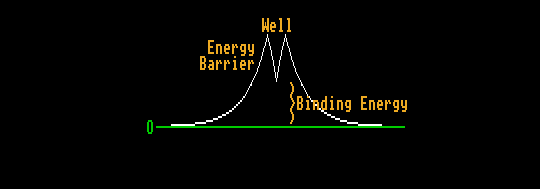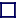### SPONTANEOUS FISSION (TUNNELLING)

The nuclei of the heavier elements have a much shallower potential well in which the nucleons reside, because the protons with like positive charge have greater effect in repelling each other against the strong nuclear force holding them together. Hence, the bottom of the potential well is above the energy of two smaller nuclei at infinite distance. But the nucleus is prevented from splitting into two nuclei by the energy barrier, the strong nuclear force holding the nucleons together within the nucleus. The nucleons within the nucleus do not have sufficient kinetic energy to surmount this energy barrier. However, this large energy could be borrowed if it were borrowed for a sufficiently short time to satisfy Heisenbergs' uncertainty principle. There is a small but finite probability that this energy could be borrowed. The higher the energy barrier, the smaller this probability. This process is known as tunnelling. The two parts of the nuclei sometimes find themselves at the same height (energy) but on the opposite side of the energy barrier, having somehow travelled at the speed of light to get there. Containing like positive charges, they then repel each other, accelerating down the foot slopes of the energy barrier, releasing the difference in energy (binding energy) as kinetic energy.

This is spontaneous fission and is very much like alpha decay, except that in alpha decay, the emitted particles are very unequal in size.

The probability of fission increases as Z2/A, the fission parameter, thus spontaneous fission is less likely than alpha decay except for very heavy nuclei (Z>105). For a fission parameter equal to 37 (corresponding to Z around 94 and A about 240 or Pu-240) the SF halflife would be of order 1010 years, whereas for a value of 40 (Z about 102, A about 260 or nobelium-102) is only a year. Nobelium-102 has an actual spontaneous fission halflife of just 106ms.